Check Important Questions and Chapter Summary -https://you.tube/Real-Numbers-Class-10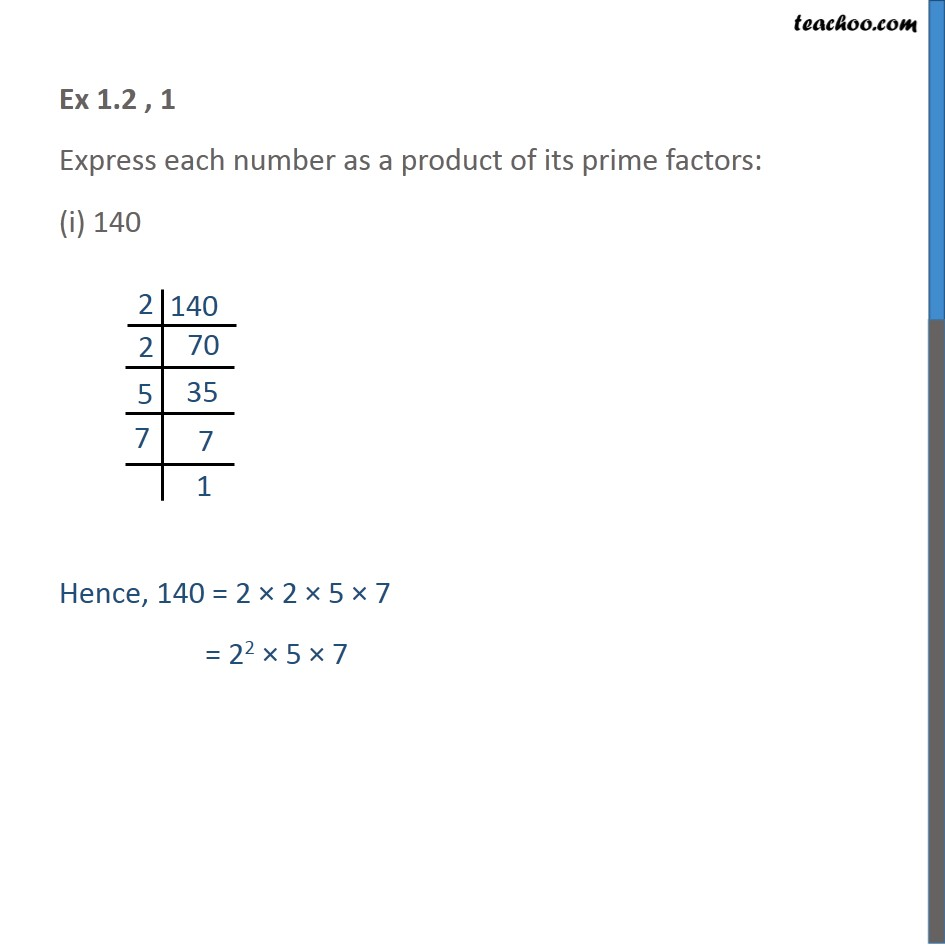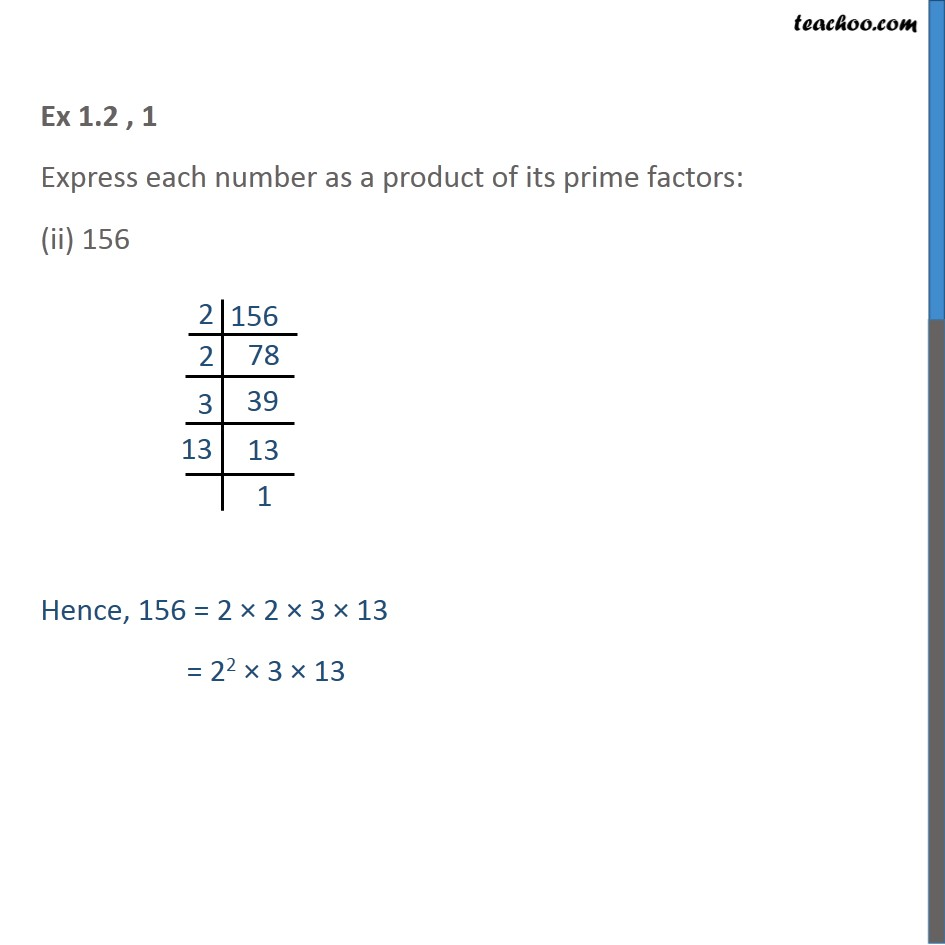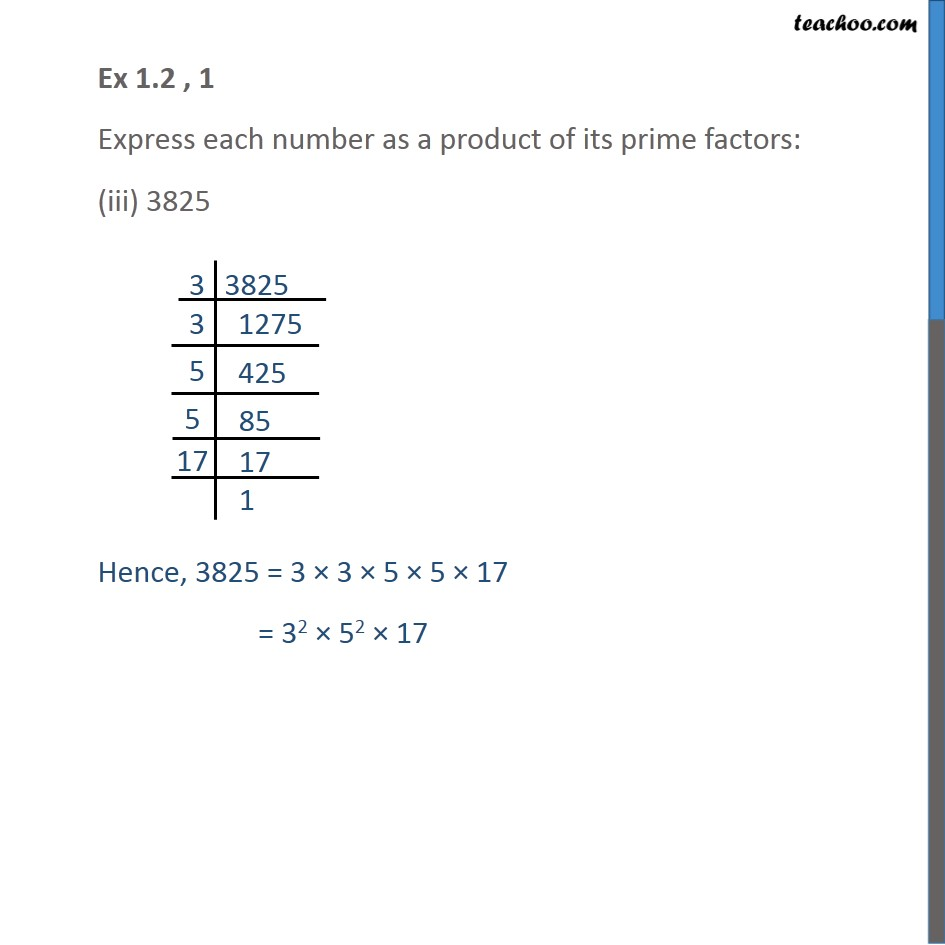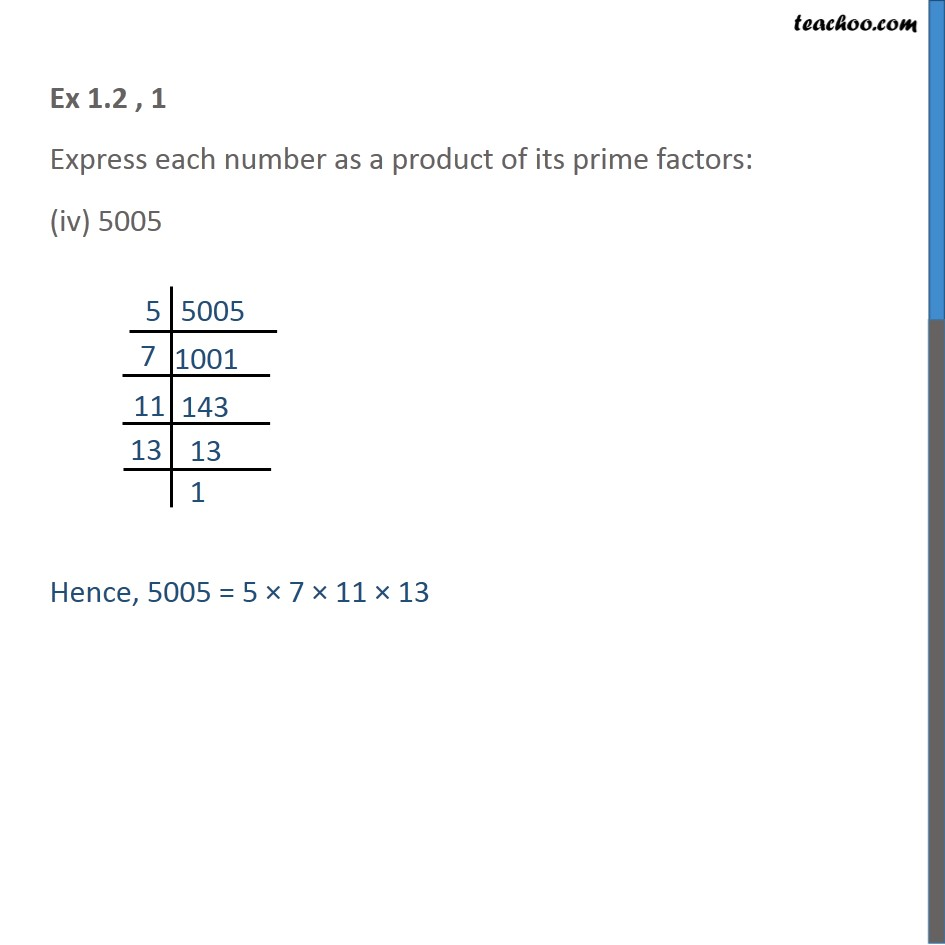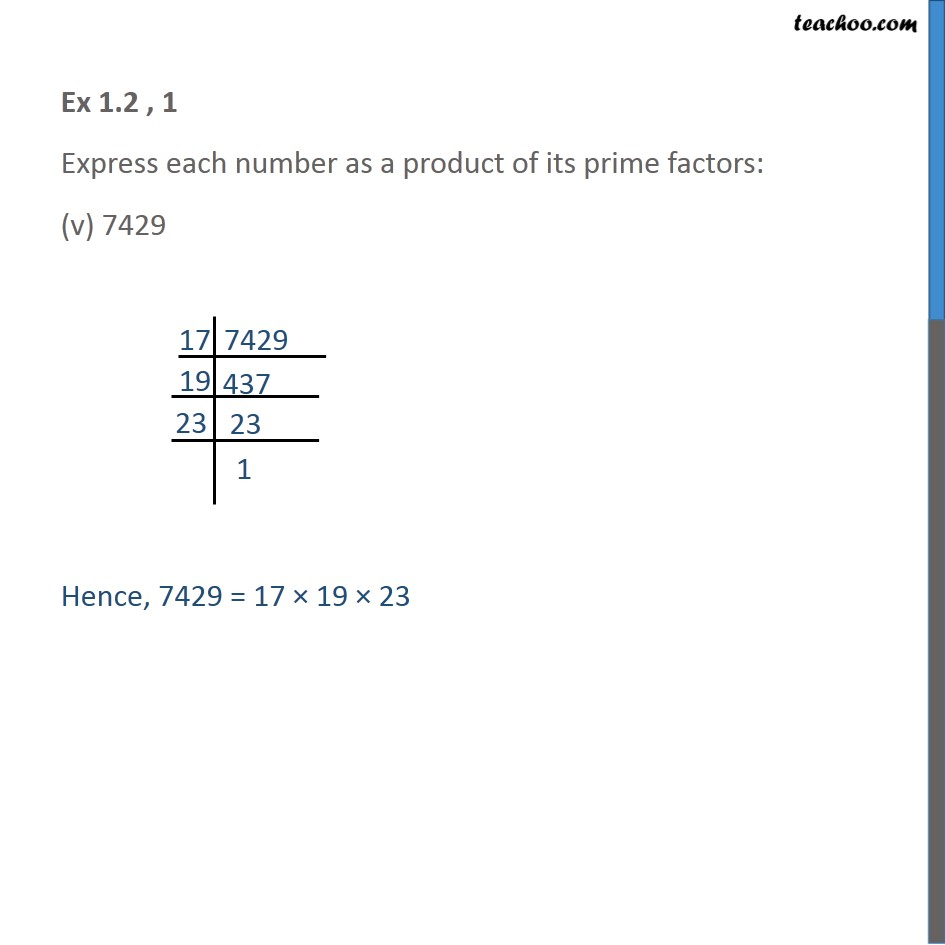1. Chapter 1 Class 10 Real Numbers
2. Concept wise
3. LCM/HCF

Transcript

Ex 1.2 , 1 Express each number as a product of its prime factors: (i) 140 Hence, 140 = 2 × 2 × 5 × 7 = 22 × 5 × 7 Ex 1.2 , 1 Express each number as a product of its prime factors: (ii) 156 Hence, 156 = 2 × 2 × 3 × 13 = 22 × 3 × 13 Ex 1.2 , 1 Express each number as a product of its prime factors: (iii) 3825 Hence, 3825 = 3 × 3 × 5 × 5 × 17 = 32 × 52 × 17 Ex 1.2 , 1 Express each number as a product of its prime factors: (iv) 5005 Hence, 5005 = 5 × 7 × 11 × 13 Ex 1.2 , 1 Express each number as a product of its prime factors: (v) 7429 Hence, 7429 = 17 × 19 × 23

LCM/HCF